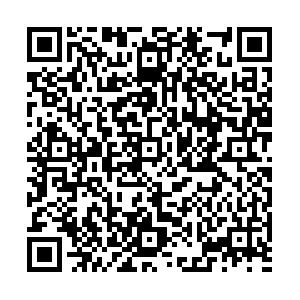Molecular picture for X0(2900) and X1(2900)• Inspired by the newly observed $X_{0}(2900)$and $X_1(2900)$states at LHCb, the $K^*\bar{D}^*$and $K\bar{D}_1$interactions are studied in the quasipotential Bethe-Salpeter equation approach combined with the one-boson-exchange model. The bound and virtual states from the interactions are searched for as poles in the complex energy plane of scattering amplitude. A bound state with $I(J^P)=0(0^+)$and a virtual state with $0(1^-)$are produced from the $K^*\bar{D}^*$interaction and $K\bar{D}_1$interaction, and can be related to the $X_{0}(2900)$and $X_1(2900)$observed at LHCb, respectively. A bound state with $I(J^P)=0(1^+)$and a virtual state with $I(J^P)=0(2^+)$are also predicted from the $K^*\bar{D}^*$interaction, with the same $\alpha$value, to reproduce the $X_{0,1}(2900)$, which can be searched for in future experiments.
••Get Citation
Jun He and Dian-Yong Chen. Molecular picture for X0(2900) and X1(2900)[J]. Chinese Physics C. doi: 10.1088/1674-1137/abeda8
Jun He and Dian-Yong Chen. Molecular picture for X0(2900) and X1(2900)[J]. Chinese Physics C.Milestone
Article Metric

Article Views(394)
Cited by(0)
Policy on re-use
To reuse of Open Access content published by CPC, for content published under the terms of the Creative Commons Attribution 3.0 license (“CC CY”), the users don’t need to request permission to copy, distribute and display the final published version of the article and to create derivative works, subject to appropriate attribution.
通讯作者: 陈斌, bchen63@163.com
• 1.

沈阳化工大学材料科学与工程学院 沈阳 110142

Title:
Email:

Molecular picture for X0(2900) and X1(2900)

Corresponding author: Dian-Yong Chen, chendy@seu.edu.cn
• 1. Department of Physics and Institute of Theoretical Physics, Nanjing Normal University, Nanjing 210097, China
• 2. School of Physics, Southeast University, Nanjing 210094, China

Abstract: Inspired by the newly observed $X_{0}(2900)$and $X_1(2900)$states at LHCb, the $K^*\bar{D}^*$and $K\bar{D}_1$interactions are studied in the quasipotential Bethe-Salpeter equation approach combined with the one-boson-exchange model. The bound and virtual states from the interactions are searched for as poles in the complex energy plane of scattering amplitude. A bound state with $I(J^P)=0(0^+)$and a virtual state with $0(1^-)$are produced from the $K^*\bar{D}^*$interaction and $K\bar{D}_1$interaction, and can be related to the $X_{0}(2900)$and $X_1(2900)$observed at LHCb, respectively. A bound state with $I(J^P)=0(1^+)$and a virtual state with $I(J^P)=0(2^+)$are also predicted from the $K^*\bar{D}^*$interaction, with the same $\alpha$value, to reproduce the $X_{0,1}(2900)$, which can be searched for in future experiments.

HTML关注分享DownLoad:  Full-Size Img  PowerPoint